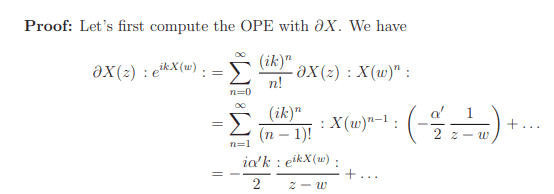# Proving that e^{ikx} is primary with weight (h=\hbar = \alpha k^2/4)

LCSphysicist
Homework Statement:
I can't understand the line of reasoning used by David Tong (on its lectures of CFT).
Relevant Equations:
.'Where

##:## really means normal ordered, in the sense that ##:A(w)B(z): = \lim_{w \to z} \left ( A(w)B(z) - \langle A(w)B(z) \rangle \right )##

##\partial X(z) = \frac{\partial X(z)}{\partial z}##

How do we go form the first line to the second one?? I am not understanding it!

$$\partial X(z) : X(w)^n : = \partial X(z) : X(w)^{n-1} X(w) :$$
Then, for some reason

$$\partial X(z) : X(w)^{n-1} X(w) : \rightarrow n X(w)^{n-1} :\partial X(z) X(w):$$

Since

$$: \partial X(z) X(w) = \frac{-\alpha'}{2 (z-w)}$$

We got the answer, but how?

•